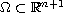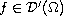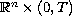Electron. J. Diff. Eqns., Vol. 2009(2009), No. 91, pp. 1-17.

### Distribution-valued weak solutions to a parabolic problem arising in financial mathematics Michael Eydenberg, Maria Cristina Mariani

Abstract:
We study distribution-valued solutions to a parabolic problem that arises from a model of the Black-Scholes equation in option pricing. We give a minor generalization of known existence and uniqueness results for solutions in bounded domainsto give existence of solutions for certain classes of distributions. We also study growth conditions for smooth solutions of certain parabolic equations onthat have initial values in the space of distributions.

Submitted September 10, 2008. Published July 30, 2009.
Math Subject Classifications: 35K10, 35D30, 91B28.
Key Words: Weak solutions; parabolic differential equations; Black-Scholes type equations.

Show me the PDF file (298 KB), TEX file, and other files for this article.

 Michael Eydenberg Department of Mathematical Sciences New Mexico State University Las Cruces, NM 88003-8001, USA email: mseyden@nmsu.edu María Cristina Mariani Department of Mathematical Sciences University of Texas, El Paso, Bell Hall 124 El Paso, Texas 79968-0514, USA email: mcmariani@utep.edu